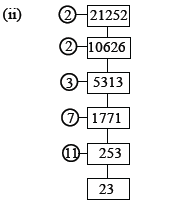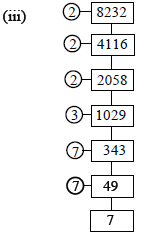CBSE Class 10 Mathematics Real Numbers Worksheet Set B

Read and download free pdf of CBSE Class 10 Mathematics Real Numbers Worksheet Set B. Students and teachers of Class 10 Real Numbers can get free printable Worksheets for Class 10 Real Numbers in PDF format prepared as per the latest syllabus and examination pattern in your schools. Standard 10 students should practice questions and answers given here for Real Numbers in Grade 10 which will help them to improve your knowledge of all important chapters and its topics. Students should also download free pdf of Class 10 Real Numbers Worksheets prepared by school teachers as per the latest NCERT, CBSE, KVS books and syllabus issued this academic year and solve important problems provided here with solutions on daily basis to get more score in school exams and tests

Real Numbers Worksheet for Class 10

Class 10 Real Numbers students should refer to the following printable worksheet in Pdf in standard 10. This test paper with questions and answers for Grade 10 Real Numbers will be very useful for exams and help you to score good marks

Class 10 Real Numbers Worksheet Pdf

REAL NUMBERS

Q.- Find the prime factors of :

(i) 540   (ii) 21252    (iii) 82325 is a prime number and so cannot be further divided by any prime number
540 = 2 × 2 × 3 × 3 × 3 × 5 = 22 × 33 ×521252 = 2 × 2 × 3 × 7 × 11 × 23
= 22 × 3 × 11 × 7 × 23∴ 8232 = 2 × 2 × 2 × 3 × 7 × 7 × 7

= 23 × 3 × 73.

Q.- Find the missing numbers a, b and c in the following factorisation:Can you find the number on top without finding the other ?

Sol. c = 17 × 2 = 34
b = c × 2 = 34 × 2 = 68 and
a = b × 2 = 68 × 2 = 136
i.e., a = 136, b = 68 and c = 34. (Ans)

Yes, we can find the number on top without finding the others.
Reason: The given numbers 2, 2, 2 and 17 are the only prime factors of the number on top and so the number on top = 2 × 2 × 2 × 17 = 136

Q.- Given that H.C.F. (306, 657) = 9, find L.C.M. (306, 657)

Sol. H.C.F. (306, 657) = 9 means H.C.F. of 306 and 657 = 9
Required L.C.M. (306, 657) means required L.C.M. of 306 and 657.
For any two positive integers;
their L.C.M. =Product of the numbers / TheirH.C.F.
i.e., L.C.M. (306, 657) =306× 657/9
= 22,338.

Q.- Given that L.C.M. (150, 100) = 300, find H.C.F. (150, 100)

Sol. L.C.M. (150, 100) = 300
=> L.C.M. of 150 and 100 = 300
Since, the product of number 150 and 100
= 150 × 100
And, we know :
H.C.F. (150, 100) = Product of 150 and 100/L.C.M.(150,100)
=150×100/300
= 50.

Q.- The H.C.F. and L.C.M. of two numbers are 12 and 240 respectively. If one of these numbers is 48; find the other numbers.

Sol. Since, the product of two numbers
= Their H.C.F. × Their L.C.M.
=> One no. × other no. = H.C.F. × L.C.M.
=> Other no. =12×240 / 48 = 60.

Q.- Explain why 7 × 11 × 13 + 13 and 7 × 6 × 5 × 4 × 3 + 5 are composite numbers.

Sol. Since,
7 × 11 × 13 + 13 = 13 × (7 × 11 + 1)
= 13 × 78 = 13 × 13 × 3 × 2;

that is, the given number has more than two factors and it is a composite number.

Similarly, 7 × 6 × 5 × 4 × 3 + 5
= 5 × (7 × 6 × 4 × 3 + 1)
= 5 × 505 = 5 × 5 × 101
=> The given no. is a composite number.

Very Short Questions-

Q.- State whether the given statement is true or false :

(i) The sum of two rationals is always rational
Ans-True

(ii) The product of two rationals is always rational
Ans-True

(iii) The sum of two irrationals is an irrational.
Ans-False

(iv) The product of two irrationals is an irrational
Ans-False

(v) The sum of a rational and an irrational is irrational
Ans-True

(vi) The product of a rational and an irrational is irrational
Ans-True

Q.- Classify the following numbers as rational or irrational :

(i) 22/7
Ans-Rational

(ii) 3.1416
Ans-Rational

(iii) π
Ans-Irrational

(iv) 3.142857
Ans-Rational

(v) 5.636363......
Ans-Rational

(vi) 2.040040004......
Ans-Irrational

(vii) 1.535335333....
Ans-Irrational

(viii) 3.121221222...
Ans-Irrational

(ix) √21
Ans-Irrational

(x) 3√3
Ans-Irrational

Q.- Without actual divison, show that each of the following rational numbers is a terminating decimal. Express each in decimal form :

(i) 23/(23 × 52)
Ans-0.115

(ii) 24/125
Ans-0.192

(iii)17/320
Ans-0.053125

(iv)171/800
Ans-0.21375

(v)15/1600
Ans-0.009375

(vi)19/3125
Ans-0.00608

Q.- A number when divided by 61 gives 27 as quotient and 32 as remainder. Find the number.
Ans-1679

Q.- By what number should 1365 be divided to get 31 as quotient and 32 as remainder ?
Ans-43

Q.- Using Euclid's algorithm, find the HCF of
(i) 405 and 2520
(ii) 504 and 1188
(iii) 960 and 1575
Ans-(i) 45   (ii) 36   (iii) 15

More question-

Q1. What is the H.C.F of the smallest composite number and the smallest prime number?

Q2. If 'p' is a prime number then what is the L.C.M of p , p2 , p3 ?

Q3. Two positive integers 'p' and 'q' can be expressed as p=ab2 and q=a2b , a and b are prime numbers . What is the L.C.M of ' p' and 'q' ?

Q4. Show that n2 -1 is divisible by 8 , if 'n' is an odd positive integer ?

Q5. Prove that n2 - n is divisible by 2 for every positive integer ' n' ?

Q6. Show that one and only one out of n , n+2 or n+4 is divisible by 3 , where n is any positive integer ?

Q7. Prove that one of every three consecutive positive integers is divisible by 3 ?

Q8. Find the H.C.F of 65 and 117 and express it in the form 65m+117n ?

Q9. If the H.C.F of 210 and 55 is expressible in the form of 210*5 + 55y , find 'y' ?

Q10. Find the largest positive integer that will divide 398, 436 and 542 leaving remainders 7, 11 and 15 respectively .

Q11. Find the greatest number of six digits exactly divisible by 24 , 15 and 36 ?

Q12. Three sets of English , Hindi and Mathematics books have to be stacked in such a way that all the books are stored topic wise and the height of each stack is the same . The number of English books is 96 , the number of Hindi books is 240 and the number of Mathematics books is 336 . Assuming that the books are of same thickness , determine the number of stacks of English , Hindi and Mathematics books ?

Q13. Two brand of chocolates are available in packs of 24 and 15 respectively . If I need to buy an equal number of chocolates of both kinds , what is the least number of boxes of each kind I would need to buy?

Q14. Prove that √2 + √5 is irrational .

Q15. Using Euclid's Division Algorithm , find whether the pair of numbers 847 and 2160 are co-prime or not .

1) 2 2) P3 3) a2b2 10) 17 11) 999720 12) 2,5,7 13) 5,8

Tags:

Click for more Real Numbers Study Material
 CBSE Class 10 Mathematics Real Numbers Worksheet Set H CBSE Class 10 Mathematics Real Numbers Worksheet Set I CBSE Class 10 Mathematics Real Numbers Worksheet Set C CBSE Class 10 Mathematics Real Numbers Worksheet Set J CBSE Class 10 Mathematics Real Numbers Worksheet Set E CBSE Class 10 Mathematics Real Numbers Worksheet Set F CBSE Class 10 Mathematics Real Numbers Worksheet Set G CBSE Class 10 Mathematics Real Numbers Worksheet Set K CBSE Class 10 Mathematics Real Numbers Worksheet Set A CBSE Class 10 Mathematics Real Numbers Worksheet Set B CBSE Class 10 Mathematics Real Numbers Worksheet Set D

Latest NCERT & CBSE News

Read the latest news and announcements from NCERT and CBSE below. Important updates relating to your studies which will help you to keep yourself updated with latest happenings in school level education. Keep yourself updated with all latest news and also read articles from teachers which will help you to improve your studies, increase motivation level and promote faster learning

CBSE OMR Sheet Guidelines for Term 1

You are aware that the CBSE will be using OMR for the first time for assessment of both the Classes-X and XII in Term-I examinations. Hence, there is a need that all the students appearing in the Term-l examinations and the schools sponsoring these students should have...

All India Children Educational Audio Video Festival

The Central Institute of Educational Technology (CIET), a constituent unit of National Council of Educational Research and Training (NCERT), is inviting entries for the 26th All India Children’s Educational Audio Video Festival (AICEAVF). This festival showcases the...

Surya Namaskar Project on 75th Anniversary of Independence Day

Ministry of Education, Govt of India vide letter No. F.No. 12-5/2020-IS-4 dated 16.12.2021 has intimated that under the banner Azadi ka Amrit Mahotsav the National Yogasanasports Federation has decided to run a project of 750 million Surya Namaskar from 01 January 2022...

MCQ Question based CBSE examination

For 2021-22 CBSE has launched MCQ question-based examination for Term 1 & Term 2 board examinations. The entire syllabus has been divided into two parts each including 50% of the entire syllabus. To score well, students must practice as per the new CBSE term-wise...

How To Solve Unseen Passages In English

Unseen passages may contain one or many paragraphs. This is one of the important yet easy parts for a student to get marks. Students should thoroughly study and understand the passage to answer the related questions. The unseen passages are there just to test the...